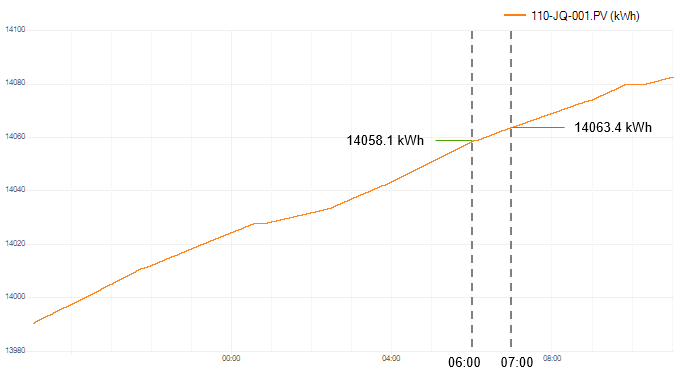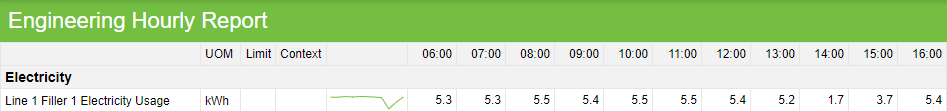Follow

# How Time Periods Transform Data into Information

Flow transforms this detailed data into context-rich information by aggregating it into time and event periods. Let’s consider the Filler 1 Electricity (kWh) totalizer shown below. Its data is logged every second, but we’re only interested in the number of kWh consumed every hour. If we “overlay” hourly time periods onto this detailed trend, we can easily summarize how many kWh were used in each hour.For the hour starting 06:00 and ending 07:00, the kWh consumed can be calculated as:

14063.4(07:00)– 14058.1(06:00)= 5.3 kWh

By repeating this process, a simple summary report of the kWh used every hour can be produced as follows:The process of summarizing data in Flow is known as data retrieval and aggregation. In the kWh totalizer example, the data is retrieved from a data source for each time period and then aggregated to provide a single piece of information for that time period (i.e. a single value for the hour starting at 06:00).

Flow makes use of different aggregation methods, depending on the detailed source data and how you want the summarized information to be presented. In the kWh totalizer example, Flow used a Counter aggregation method.

Other aggregation methods include Minimum, Maximum, Range, Average, Sum, First, Last, Delta, Count, Variance, Standard Deviation and Time in State